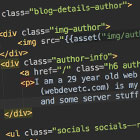## General Webdev and Programming Stuff: Push vs Pop vs Shift vs Unshift

These four functions - `push()`, `pop()`, `shift()` and `unshift` - are common in many programming languages. They are used for adding and removing elements to arrays (either from the start or end).

The specific implementations of each function will vary between languages (it might be `push(\$array,'new item')`, `\$array.push('new_item')`, etc. But the basic idea is normally the same:

End of array = the last item (if the array has 5 elements, then index #4).
Start = first item, so item #0

### Adding to arrays: push() and pop()

#### push

`push()` will add (push) an item to the end of an array.

In php, it is `array_push(&array, \$new_el)`

In javascript it is `some_array_variable.push(new_el)`

#### unshift

`unshift()` will add (prepend) an item to the start of an array.

In php, it is `array_unshift(&array, \$new_el)`

In javascript, it is `some_array_var.unshift(add_this_obj)`

### Removing from arrays: pop() and shift()

In most languages these will remove the item from an array, then return that removed item.

#### pop

`pop()` will remove (pop) an item from the end of an array.

In php, it is `array_pop(&\$array)`

In javascript, it is `some_array.pop()`

#### shift

`shift()` will remove (shift) an item from the start of an array.

In php, it is `array_shift(&array)`)

In javascript, it is `another_array.shift()`

## Some examples in PHP of adding/removing items from the start and end of arrays:

PHP Push example - Add to the end

`\$stack = array("orange", "banana", "apple", "raspberry");array_push(\$stack, "NEW_ITEM");print_r(["stack"=>\$stack,]);`
```Array
(
 => orange
 => banana
 => apple
 => raspberry
 => NEW_ITEM
)```

PHP Unshift example - add to the start

`\$stack = array("orange", "banana", "apple", "raspberry");array_unshift(\$stack, "NEW_ITEM");print_r(\$stack);`
```Array
(
 => NEW_ITEM
 => orange
 => banana
 => apple
 => raspberry
)```

PHP Pop example - remove from the end

`\$stack = array("orange", "banana", "apple", "raspberry");\$removed = array_pop(\$stack);print_r(["stack"=>\$stack,'removed_item_of_fruit' => \$removed]);`
```Array
(
[stack] => Array
(
 => orange
 => banana
 => apple
)

[removed_item_of_fruit] => raspberry
)```

PHP Shift example - remove from the start

`\$stack = array("orange", "banana", "apple", "raspberry");\$fruit = array_shift(\$stack);print_r(\$stack);`
```Array
(
[stack] => Array
(
 => banana
 => apple
 => raspberry
)

[shifted_item_of_fruit] => orange
)```webdevetc

I am a 29 year old backend web developer from London, mostly focusing on PHP and Laravel lately. This (webdevetc.com) is my blog where I write about some web development topics (PHP, Laravel, Javascript, and some server stuff). contact me here.

### More...

##### Or see other languages/frameworks:
PHP Laravel Composer Apache CentOS and Linux Stuff WordPress General Webdev and Programming Stuff JavaScript